# MP Board Class 10th Maths Solutions Chapter 6 Triangles Ex 6.6

In this article, we will share MP Board Class 10th Maths Book Solutions Chapter 6 Triangles Ex 6.6 Pdf, These solutions are solved subject experts from the latest edition books.

## MP Board Class 10th Maths Solutions Chapter 6 Triangles Ex 6.6

Question 1.
In the figure, PS is the bisector of ∠QPR of ∆PQR. Prove that $$\frac{Q S}{S R}=\frac{P Q}{P R}$$.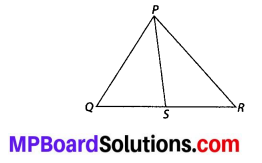Solution:
We have, ∆PQR in which PS is the bisector of ∠QPR.
∴ ∠QPS = ∠RPS
Let us draw RT || PS to meet QP produced at T, such that
∠1 = ∠RPS [Alternate angles]
Also, ∠3 = ∠QPS [Corresponding angles]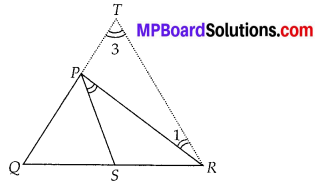But ∠RPS = ∠QPS [Given]
∴ ∠1 = ∠3
∴ PT = PR
Now, in ∆QRT, PS || RT [By construction]
∴ Using the Basic Proportionality Theorem, we have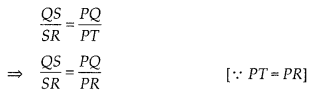Question 2.
In the figure, D is a point on hypotenuse AC of ∆ABC, such that BD ⊥ AC, DM ⊥ BC and DN ⊥ AB. Prove that
(i) DM2 = DN.MC
(ii) DN2 = DM.AN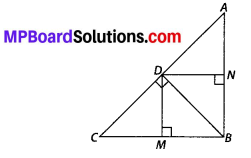Solution: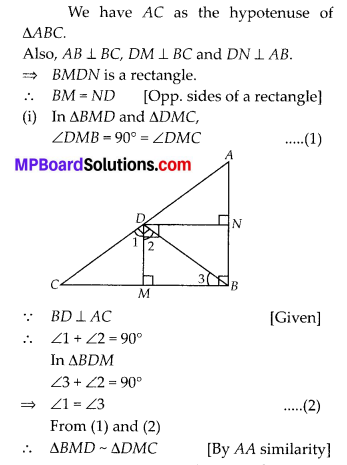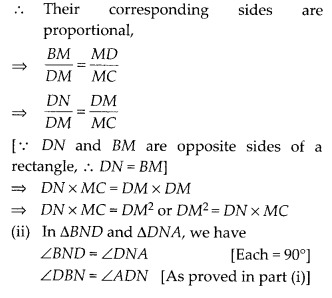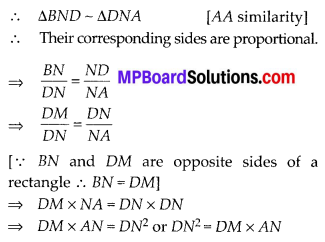Question 3.
In the figure, ABC is a triangle in which ∠ABC > 90° and AD ⊥ CB produced. Prove that AC2 – AB2 + BC2 + 2BC.BD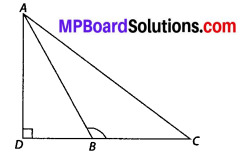Solution:
∆ABC is a triangle in which ∠ABC > 90° and AD ⊥ CB produced.
∵ In ∆ADB, ∠D = 90°
∴ Using Pythgoras Theorem, we have
AB2 = AD2 + DB2 ….. (1)
In right ∆ADC, ∠D = 90°
∴ Using Pythagoras Theorem, we have
= AD2 + [BD + BC]2
= AD2 + [BD2 + BC2 + 2BD.BC]
⇒ AC2 = [AD2 + DB2] + BC2 + 2BC – BD
⇒ AC2 = AB2 + BC2 + 2BC – BD [From (1)] Thus, AC2 = AB2 + BC2 + 2 BC.BDQuestion 4.
In the figure, ABC is a triangle in which ∠ABC < 90° and AD ⊥ BC. Prove that AC2 = AB2 + BC2 – 2BC.BD.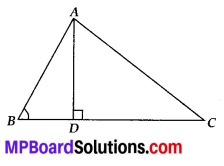Solution:
We have ∆ABC in which ∠ABC < 90°
In right ∆ADB, ∠D = 90°
Using Pythagoras Theorem, we have
AB2 = AD2 + BD2 …… (1)
Also in right ∆ADC, ∠D = 90°
Using Pythagoras Theorem, we have AC2 = AD2 + DC2
= AD2 + [BC – BD]2 = AD2 + [BC2 + BD2 – 2BC.BD]
= [AD2 + BD2] + BC2 – 2BC.BD = AB2 + BC2 – 2BC.BD [From (1)]
Thus, AC2 = AB2 + BC2 – 2BC.BD, which is the required relation.

Question 5.
In the figure, AD is a median of triangle ABC and AM ⊥ BC. Prove that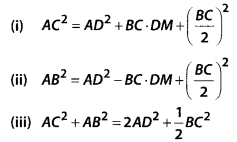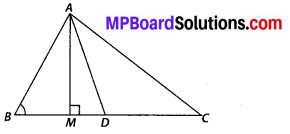Solution: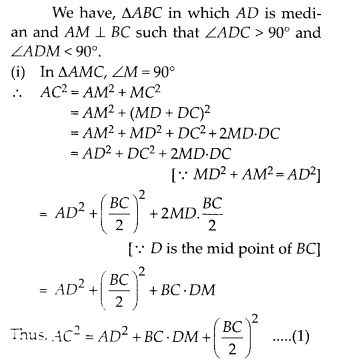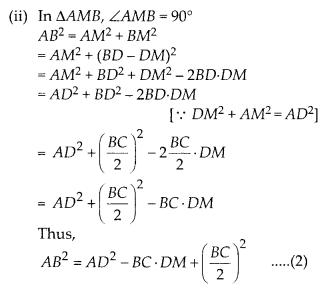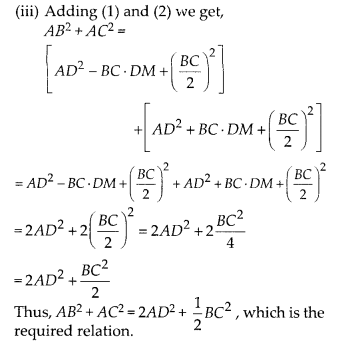Question 6.
Prove that the sum of the squares of the diagonals of parallelogram is equal to the sum of the squares of its sides.
Solution:
We have a parallelogram ABCD.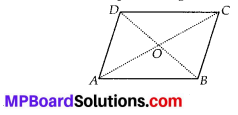AC and BD are the diagonals of parallelogram ABCD.
∵ Diagonals of a parallelogram bisect each other.
∴ O is the mid-point of AC and BD.
Now, in ∆ABC, BO is a median.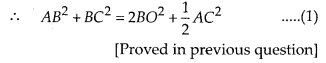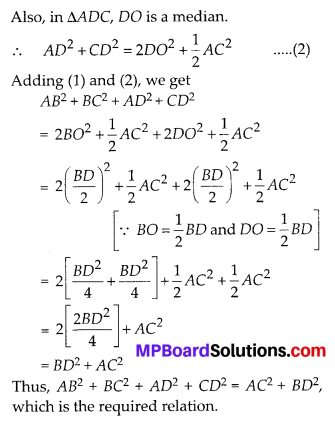Question 7.
In the figure, two chords AB and CD intersect each other at the point P. Prove that:
(i) ∆APC – ∆DPB
(ii) AP.PB = CP.DPSolution:
We have two chords AB and CD of a circle. AB and CD intersect at P.
(i) In ∆APC and ∆DPB,
∴ ∠APC = ∠DPB ….. (1)
[Vertically opp. angles]
∠CAP = ∠BDP …… (2)
[Angles in the same segment]
From (1) and (2) and using AA similarity, we have
∆APC ~ ∆DPB

(ii) Since, ∆APC ~ ∆DPB [As proved above]
∴ Their corresponding sides are proportional,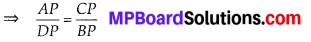⇒ AP.BP = CP.DP, which is the required relation.

Question 8.
In the figure, two chords AB and CD of a circle intersect each other at the point P (when produced) outside the circle. Prove that
(i) ∆PAC – ∆PDB
(ii) PA.PB = PC.PDSolution:
We have two chords AB and CD when produced meet outside the circle at P.
(i) Since in a cyclic quadrilateral, the exterior angle is equal to the interior opposite angle,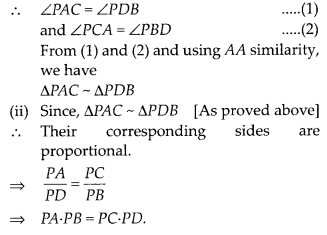Question 9.
In the figure, D is a point on side BC of ∆ABC such that $$\frac{B D}{C D}=\frac{A B}{A C}$$. Prove that AD is the bisector of ∠BAC.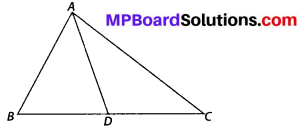Solution:
Let us produce BA to E such that AE = AC
Join EC.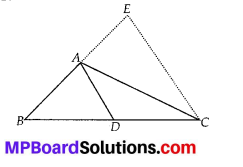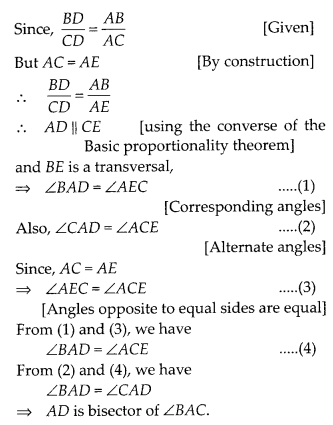Question 10.
Nazima is fly fishing in a stream. The tip of her fishing rod is 1.8 m above the surface of the water and the fly at the end of the string rests on the water 3.6 m away and 2.4 m from a point directly under the tip of the rod. Assuming that her string (from the tip of her rod to the fly) is taut, how much string does she have out (see figure)? If she pulls in the string at the rate of 5 cm per second, what will be the horizontal distance of the fly from her after 12 seconds ?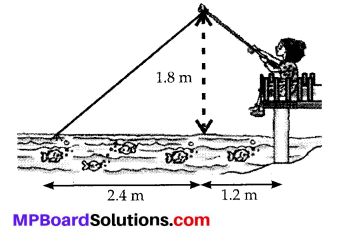Solution:
Let us find the length of the string that Nazima has out.
In right ∆OAB, OB2 = OA2 + AB2
∴ OB2 = (2.4)2 + (1.8)2
⇒ OB2 = 5.76 + 3.24 = 9.00
$$\Rightarrow \quad O B=\sqrt{9.00}=3 \mathrm{m}$$
i.e., Length of string she has out = 3 m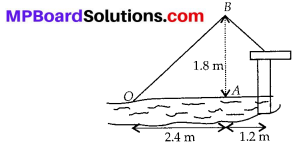Since, the string is pulled in at the rate of 5 cm/sec,
∴ Length of the string pulled in 12 seconds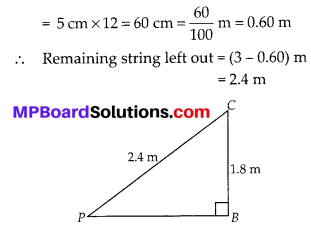In the ∆PBC, let PB be the required horizontal distance of fly.
Since, PB2 = PC2 – BC2 [By Pythagoras theorem]
∴ PB2 = (2.4)2 – (1.8)2 = 5.76 – 3.24 = 2.52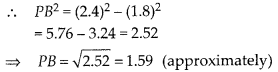Thus, the horizontal distance of the fly from Nazima after 12 seconds
= (1.59 + 1.2) m (approximately)
= 2.79 m (approximately)